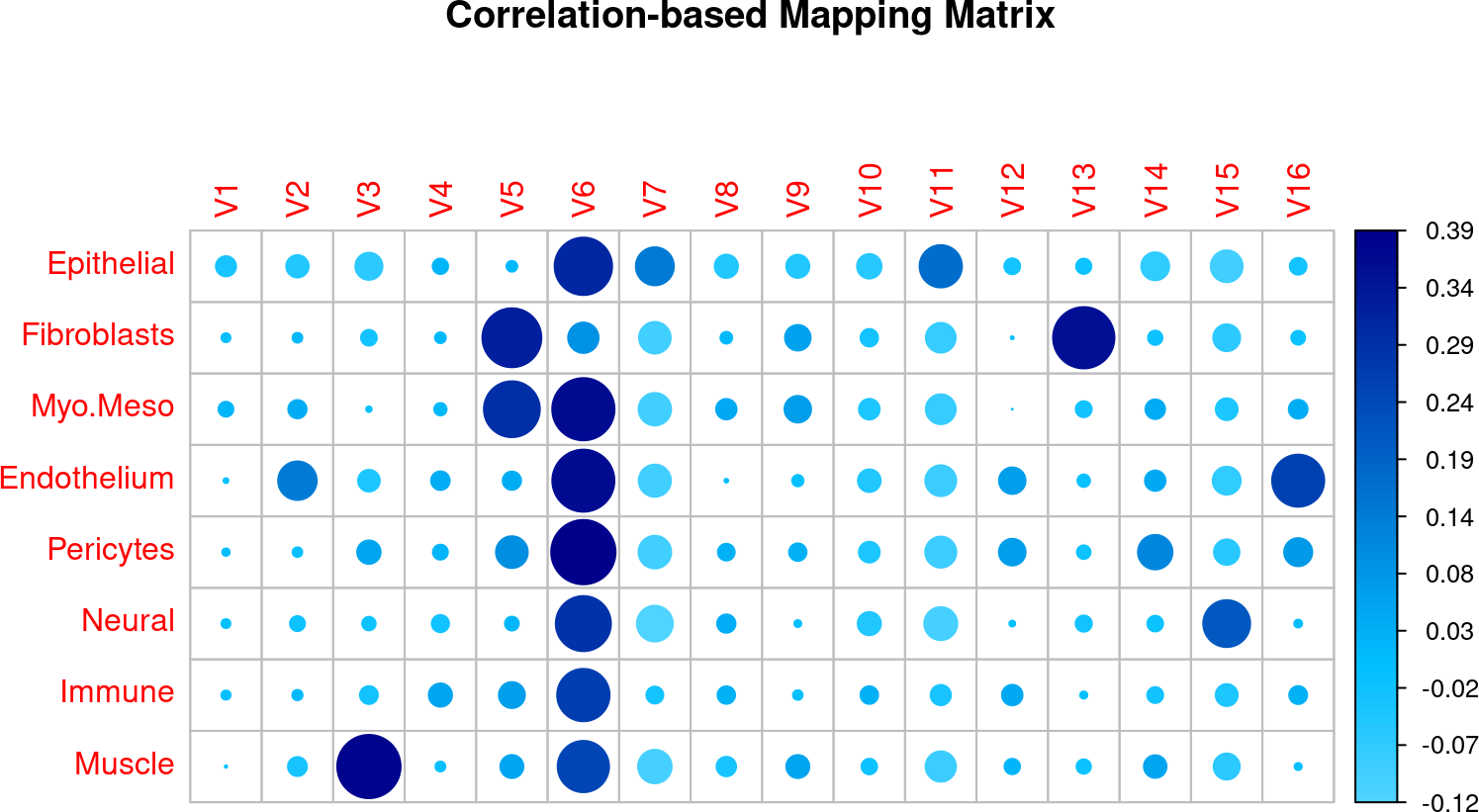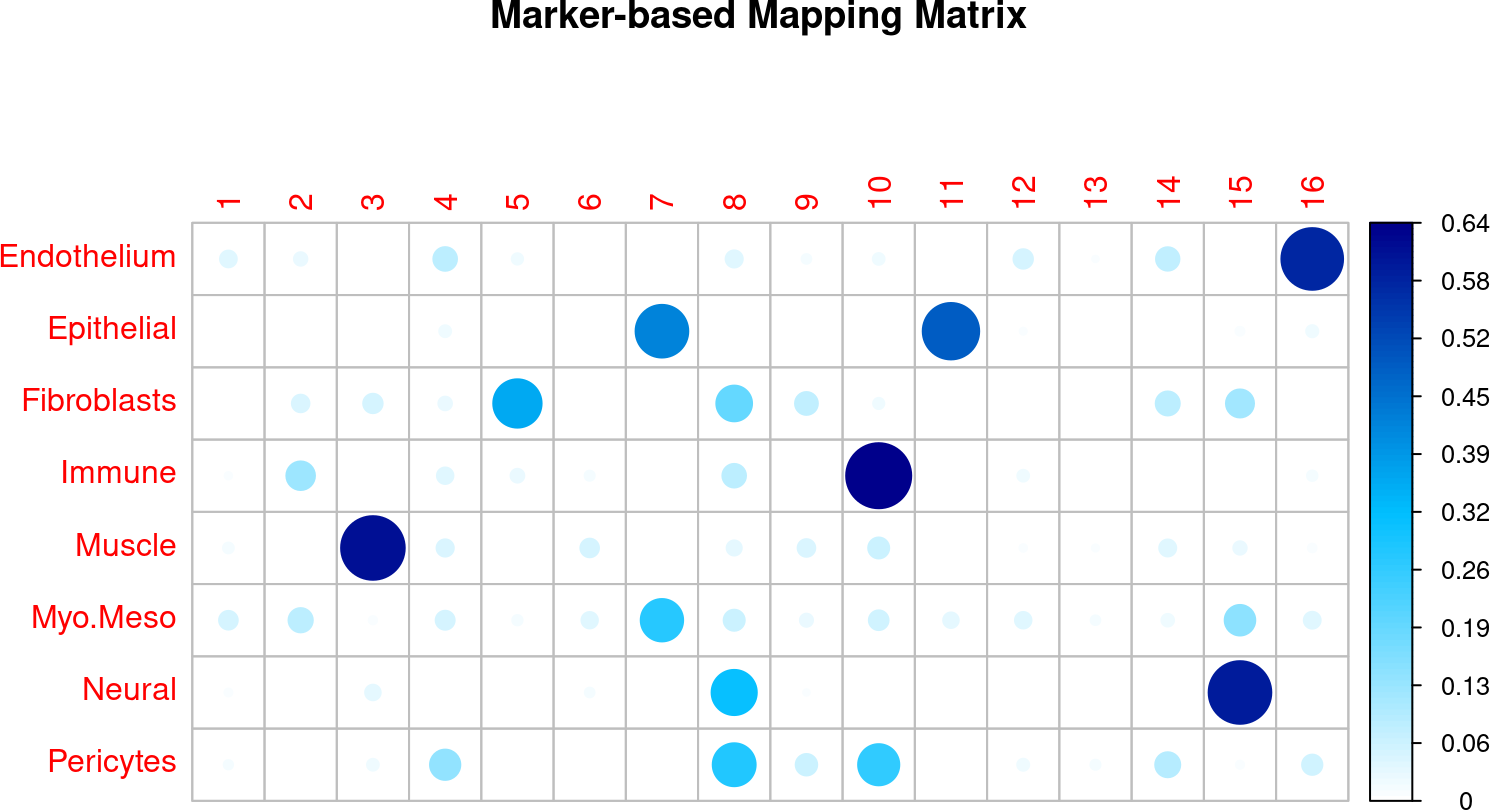# 1 Introduction

RETROFIT is a statistical method for reference-free deconvolution of spatial transcriptomics data to estimate cell type mixtures. In this Vignette, we will estimate cell type composition of a Colon dataset. We will annotate cell types using marker gene lists as well as single cell reference. We will also reproduce some of the results from the paper for illustration.

# 2 Package Installation and other requirements

Install and load the packages using the following steps:

``````if(!requireNamespace("BiocManager", quietly = TRUE))
install.packages("BiocManager")
BiocManager::install("retrofit")``````
``library(retrofit)``

# 3 Spatial Transcriptomics Data

First load the Colon ST data from fetal 12 PCW tissue, using the following command:

``````data("vignetteColonData")
X = vignetteColonData\$a3_x``````

# 4 Reference-free Deconvolution

Initialize the required parameters for deconvolution. - iterations: Number of iterations (default = 4000) - L: Number of components required

``````iterations  = 10
L           = 20``````

After initialization, run retrofit on the data (X) as follows:

``result = retrofit::decompose(X, L=L, iterations=iterations, verbose=TRUE)``
``````##  "iteration: 1 0.138 Seconds"
##  "iteration: 2 0.139 Seconds"
##  "iteration: 3 0.139 Seconds"
##  "iteration: 4 0.141 Seconds"
##  "iteration: 5 0.147 Seconds"
##  "iteration: 6 0.164 Seconds"
##  "iteration: 7 0.14 Seconds"
##  "iteration: 8 0.142 Seconds"
##  "iteration: 9 0.155 Seconds"
##  "iteration: 10 0.144 Seconds"
##  "Iteration mean:  0.145  seconds  total:  1.45  seconds"``````
``````H   = result\$h
W   = result\$w
Th  = result\$th``````

After deconvolution of ST data, we have our estimates of W (a matrix of order G x L), H (a matrix of order L x S) and Theta (a vector of L components). Here, we are using number of iterations as 20 for demonstration purposes. For reproducing results in the paper, we need to run RETROFIT for iterations = 4000. The whole computation is omitted here due to time complexity (> 10min). We will load the results from 4000 iterations for the rest of the analysis.

``````H   = vignetteColonData\$a3_results_4k_iterations\$decompose\$h
W   = vignetteColonData\$a3_results_4k_iterations\$decompose\$w
Th  = vignetteColonData\$a3_results_4k_iterations\$decompose\$th``````

Above results are obtained by running the code below.

``````iterations = 4000
set.seed(1)
result = retrofit::decompose(x, L=L, iterations=iterations)``````

Next, we need to annotate the components, to get the proportion of K cell types. We can do this in two ways: (a) using an annotated single cell reference or (b) using the known marker genes.

# 5 Cell-type Annotation via annotated single-cell reference

Here, we will annotate components using single-cell reference. Load the single-cell reference data:

``sc_ref = vignetteColonData\$sc_ref``

This file contains average gene expression values for K=8 cell types. Run the following command to get K cell-type mixtures from the ST data X:

``````K = ncol(sc_ref) # number of cell types
result    = annotateWithCorrelations(sc_ref, K, W, H)
H_sc      = result\$h
W_sc      = result\$w
H_sc_prop = result\$h_prop
W_sc_prop = result\$w_prop``````

We assign components to the cell type it has maximum correlation with as shown in figure above. Finally, cell-type proportions for each spot in the ST data (X) are stored in H_mark_prop of order K x S. You can verify that the mappings from both methods are largely similar.

``````W_norm <- W
for(i in 1:nrow(W)){
W_norm[i,]=W[i,]/sum(W[i,])
}

corrplot::corrplot(stats::cor(sc_ref, W_norm),
is.corr=FALSE,
mar=c(0,0,1,0),
col = colorRampPalette(c("white", "deepskyblue", "blue4"))(100),
main="Correlation-based Mapping Matrix")``````# 6 Cell-type Annotation via known marker genes

Here, we will annotate using known marker genes for cell types. Load the marker gene list:

``marker_ref = vignetteColonData\$marker_ref``

This file contains the list of marker genes for K = 8 cell types. Run the following command to get K cell-type mixtures from the ST data X:

``````K = length(marker_ref) # number of cell types
result      = retrofit::annotateWithMarkers(marker_ref, K, W, H)
H_mark      = result\$h
W_mark      = result\$w
H_mark_prop = result\$h_prop
W_mark_prop = result\$w_prop
gene_sums   = result\$gene_sums``````
``````corrplot::corrplot(gene_sums,
is.corr=FALSE,
mar=c(0,0,1,0),
col=colorRampPalette(c("white", "deepskyblue", "blue4"))(100),
main="Marker-based Mapping Matrix")``````We assign components to the cell type it has maximum average marker expression in, as shown in figure above. Finally, cell-type proportions for each spot in the ST data (X) are stored in H_est of order K x S.

# 7 Results and visualization

Hereon, we will be reproducing some of the analysis from the paper using marker-based mapping results.

## 7.1 Figure 4A: Proportion of different cell types in the tissue.

Proportion of cell-types in this tissue:

``rowSums(H_mark_prop)/ncol(H_mark_prop)``
``````## Endothelium  Epithelial Fibroblasts      Immune      Muscle    Myo.Meso
##  0.13018273  0.13980586  0.24423669  0.09745911  0.12181790  0.10049303
##      Neural   Pericytes
##  0.09560567  0.07039902``````

## 7.2 Figure 4C: Localization of cell types with the dominant cell type in each spot

Load the coordinates for the spots in the tissue and the tissue image:

``````coords=vignetteColonData\$a3_coords
t <- grid::rasterGrob(img, width=ggplot2::unit(1,"npc"), height=ggplot2::unit(1,"npc"), interpolate = FALSE)``````

Find the cell-type with maximum proportion in each spot and plot:

``````dat=as.data.frame(cbind(coords,t(H_mark_prop)))
colnames(dat)=c(colnames(coords),rownames(H_mark_prop))

df=dat[,-c(1:3)]
df\$CellType=NA
for(i in 1:nrow(df)){
df\$CellType[i]=names(which.max(df[i,-c(1:2)]))
}
df\$CellType=factor(df\$CellType,levels=c("Epithelial", "Immune", "Myo.Meso","Muscle",
"Neural", "Endothelium", "Pericytes","Fibroblasts"))

ggplot2::ggplot(df, ggplot2::aes(x=imagerow, y=imagecol))  +
ggplot2::geom_point(size=1.8, shape=21, ggplot2::aes(fill=CellType))+
ggplot2::scale_fill_manual(values=c("#999999", "#E69F00", "#56B4E9","#009E73", "#CC79A7",
"#0072B2", "#D55E00" ,"#F0E442"),
labels=c("Epithelial", "Immune","Myofibroblasts/\nMesothelium",
"Muscle","Neural","Endothelium",
"Pericytes","Fibroblasts"))+
ggplot2::xlab("") + ggplot2::ylab("") + ggplot2::theme_classic()+
ggplot2::theme(legend.position = "none",
axis.line = ggplot2::element_blank(),
plot.margin = ggplot2::margin(-0.2, -0.2, 0.2, -1, "cm"),
axis.text.x = ggplot2::element_blank(), axis.text.y = ggplot2::element_blank(),
axis.ticks.x = ggplot2::element_blank(),  axis.ticks.y = ggplot2::element_blank())                   ``````